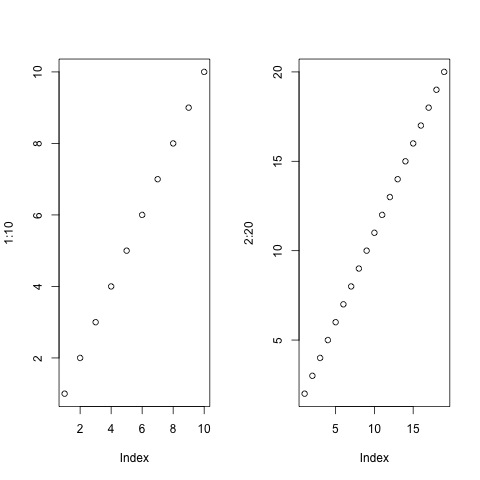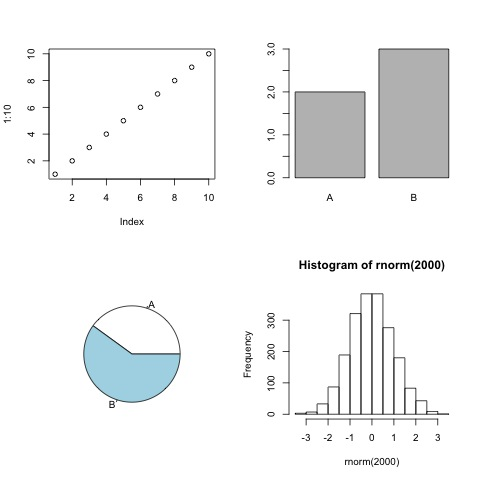## 14.2 With the console

``````# Open the file that will contain your plot (the name is up to you)
pdf(file="myplot.pdf")

# execute the plot
plot(1:10)

# Close and save the file that will contain the plot
dev.off()``````

#### Formats

R supports a variety of file formats for figures: pdf, png, jpeg, tiff, bmp, svg, ps.
They all come with their own function, for example:

``````# TIFF
tiff(file="myfile.tiff")

plot(1:10)

dev.off()

# JPEG
jpeg(file="myfile.jpeg")

plot(1:10)

dev.off()

# etc.``````

The size of the output file can be changed:

``````# Default: 7 inches (both width and height) for svg, pdf, ps.
svg(file="myfile.svg", width=8, height=12)

plot(1:10)

dev.off()

# Default: 480 pixels (both width and height) for jpeg, tiff, png, bmp.
png(file="myfile.png", width=500, height=600)

plot(1:10)

dev.off()``````

Note that pdf is the only format that supports saving several pages:

``````pdf(file="myfile_pages.pdf")

plot(1:10)
plot(2:20)

dev.off()``````
• If your file is empty: run `dev.off()` once or more to make sure there is no devices/files still open!.

#### Plot several figures in one page

You can output more than one plot per page using the par() function (`par()` sets graphical parameters) and the mfrow argument.

``````jpeg(file="myfile_multi.jpeg")

# organize the plot in 1 row and 2 columns:
# nr: number of rows
# nc: number of columns
par(mfrow=c(nr=1, nc=2))

plot(1:10)
plot(2:20)

dev.off()````````````jpeg(file="myfile_multi4.jpeg")

# organize the plot in 2 rows and 2 columns
par(mfrow=c(nr=2, nc=2))

# top-left
plot(1:10)
# top-right
barplot(table(rep(c("A","B"), c(2,3))))
# bottom-left
pie(table(rep(c("A","B"), c(2,3))))
# bottom-right
hist(rnorm(2000))

dev.off()``````HANDS-ON

Go back to the previous plots you created (if you didn’t save commands in an R script, you can refer to the History tab in the top-right panel):

• Save 1 plot of your choice in a jpeg file.
• Save 3 plots of your choice in a pdf file (one plot per page).
• Organize the same 3 plots in 1 row / 3 columns. Save the image in a png file. Play with the width (and perhaps also height) argument of `png()` until you are satisfied with the way the plot renders.
``````# Save 1 plot of your choice in a **jpeg** file.
jpeg("myboxplot.jpeg")
boxplot(chickwts\$weight ~ chickwts\$feed, las=2)
dev.off()

# Save 3 plots of your choice in a **pdf** file (one plot per page).
pdf("my3plots.pdf")
boxplot(chickwts\$weight ~ chickwts\$feed, las=2)
barplot(table(chickwts\$feed))
plot(x=Loblolly\$age, y=Loblolly\$height,
main="Age and weight of Loblolly pine trees",
pch=17,
col="red",
cex=0.4)
dev.off()

# Organize the same 3 plots in 1 row / 3 columns. Save the image in a **png** file.
png("my3plots.png", width=1000, height=350)
par(mfrow=c(1, 3))
boxplot(chickwts\$weight ~ chickwts\$feed, las=2)
barplot(table(chickwts\$feed))
plot(x=Loblolly\$age, y=Loblolly\$height,
main="Age and weight of Loblolly pine trees",
pch=17,
col="red",
cex=0.4)
dev.off()``````